## 10.8 Work and Power for Rotational Motion

### Learning Objectives

By the end of this section, you will be able to:

• Use the work-energy theorem to analyze rotation to find the work done on a system when it is rotated about a fixed axis for a finite angular displacement
• Solve for the angular velocity of a rotating rigid body using the work-energy theorem
• Find the power delivered to a rotating rigid body given the applied torque and angular velocity
• Summarize the rotational variables and equations and relate them to their translational counterparts

Thus far in the chapter, we have extensively addressed kinematics and dynamics for rotating rigid bodies around a fixed axis. In this final section, we define work and power within the context of rotation about a fixed axis, which has applications to both physics and engineering. The discussion of work and power makes our treatment of rotational motion almost complete, with the exception of rolling motion and angular momentum, which are discussed in Angular Momentum. We begin this section with a treatment of the work-energy theorem for rotation.

### Work for Rotational Motion

Now that we have determined how to calculate kinetic energy for rotating rigid bodies, we can proceed with a discussion of the work done on a rigid body rotating about a fixed axis. (Figure) shows a rigid body that has rotated through an angle $d\theta$ from A to B while under the influence of a force $\overset{\to }{F}$. The external force $\overset{\to }{F}$ is applied to point P, whose position is $\overset{\to }{r}$, and the rigid body is constrained to rotate about a fixed axis that is perpendicular to the page and passes through O. The rotational axis is fixed, so the vector $\overset{\to }{r}$ moves in a circle of radius r, and the vector $d\overset{\to }{s}$ is perpendicular to $\overset{\to }{r}.$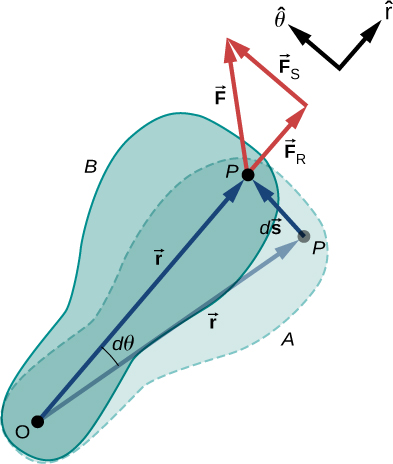Figure 10.39 A rigid body rotates through an angle $d\theta$ from A to B by the action of an external force $\overset{\to }{F}$ applied to point P.

From (Figure), we have

$\overset{\to }{s}=\overset{\to }{\theta }\,×\,\overset{\to }{r}.$

Thus,

$d\overset{\to }{s}=d(\overset{\to }{\theta }\,×\,\overset{\to }{r})=d\overset{\to }{\theta }\,×\,\overset{\to }{r}+d\overset{\to }{r}\,×\,\overset{\to }{\theta }=d\overset{\to }{\theta }\,×\,\overset{\to }{r}.$

Note that $d\overset{\to }{r}$ is zero because $\overset{\to }{r}$ is fixed on the rigid body from the origin O to point P. Using the definition of work, we obtain

$W=\int \sum \overset{\to }{F}·d\overset{\to }{s}=\int \sum \overset{\to }{F}·(d\overset{\to }{\theta }\,×\,\overset{\to }{r})=\int d\overset{\to }{\theta }·(\overset{\to }{r}\,×\,\sum \overset{\to }{F})$

where we used the identity $\overset{\to }{a}·(\overset{\to }{b}\,×\,\overset{\to }{c})=\overset{\to }{b}·(\overset{\to }{c}\,×\,\overset{\to }{a})$. Noting that $(\overset{\to }{r}\,×\,\sum \overset{\to }{F})=\sum \overset{\to }{\tau }$, we arrive at the expression for the rotational work done on a rigid body:

$W=\int \sum \overset{\to }{\tau }·d\overset{\to }{\theta }.$

The total work done on a rigid body is the sum of the torques integrated over the angle through which the body rotates. The incremental work is

$dW=(\sum _{i}{\tau }_{i})d\theta$

where we have taken the dot product in (Figure), leaving only torques along the axis of rotation. In a rigid body, all particles rotate through the same angle; thus the work of every external force is equal to the torque times the common incremental angle $d\theta$. The quantity $(\sum _{i}{\tau }_{i})$ is the net torque on the body due to external forces.

Similarly, we found the kinetic energy of a rigid body rotating around a fixed axis by summing the kinetic energy of each particle that makes up the rigid body. Since the work-energy theorem ${W}_{i}=\text{Δ}{K}_{i}$ is valid for each particle, it is valid for the sum of the particles and the entire body.

Work-Energy Theorem for Rotation

The work-energy theorem for a rigid body rotating around a fixed axis is

${W}_{AB}={K}_{B}-{K}_{A}$

where

$K=\frac{1}{2}I{\omega }^{2}$

and the rotational work done by a net force rotating a body from point A to point B is

${W}_{AB}=\underset{{\theta }_{A}}{\overset{{\theta }_{B}}{\int }}(\sum _{i}{\tau }_{i})d\theta .$

We give a strategy for using this equation when analyzing rotational motion.

### Problem-Solving Strategy: Work-Energy Theorem for Rotational Motion

1. Identify the forces on the body and draw a free-body diagram. Calculate the torque for each force.
2. Calculate the work done during the body’s rotation by every torque.
3. Apply the work-energy theorem by equating the net work done on the body to the change in rotational kinetic energy.

Let’s look at two examples and use the work-energy theorem to analyze rotational motion.

### Example

#### Rotational Work and Energy

A $12.0\,\text{N}·\text{m}$ torque is applied to a flywheel that rotates about a fixed axis and has a moment of inertia of $30.0\,\text{kg}·{\text{m}}^{2}$. If the flywheel is initially at rest, what is its angular velocity after it has turned through eight revolutions?

#### Strategy

We apply the work-energy theorem. We know from the problem description what the torque is and the angular displacement of the flywheel. Then we can solve for the final angular velocity.

#### Solution

The flywheel turns through eight revolutions, which is $16\pi$ radians. The work done by the torque, which is constant and therefore can come outside the integral in (Figure), is

${W}_{AB}=\tau ({\theta }_{B}-{\theta }_{A}).$

We apply the work-energy theorem:

${W}_{AB}=\tau ({\theta }_{B}-{\theta }_{A})=\frac{1}{2}I{\omega }_{B}^{2}-\frac{1}{2}I{\omega }_{A}^{2}.$

With $\tau =12.0\,\text{N}·\text{m},{\theta }_{B}-{\theta }_{A}=16.0\pi \,\text{rad},\,I=30.0\,\text{kg}·{\text{m}}^{2},\,\text{and}\,{\omega }_{A}=0$, we have

$12.0\,\text{N-m}(16.0\pi \,\text{rad})=\frac{1}{2}(30.0\,\text{kg}·{\text{m}}^{2})({\omega }_{B}^{2})-0.$

Therefore,

${\omega }_{B}=6.3\,\text{rad}\text{/}\text{s}.$

This is the angular velocity of the flywheel after eight revolutions.

#### Significance

The work-energy theorem provides an efficient way to analyze rotational motion, connecting torque with rotational kinetic energy.

### Example

#### Rotational Work: A Pulley

A string wrapped around the pulley in (Figure) is pulled with a constant downward force $\overset{\to }{F}$ of magnitude 50 N. The radius R and moment of inertia I of the pulley are 0.10 m and $2.5\,×\,{10}^{-3}{\text{kg-m}}^{2}$, respectively. If the string does not slip, what is the angular velocity of the pulley after 1.0 m of string has unwound? Assume the pulley starts from rest.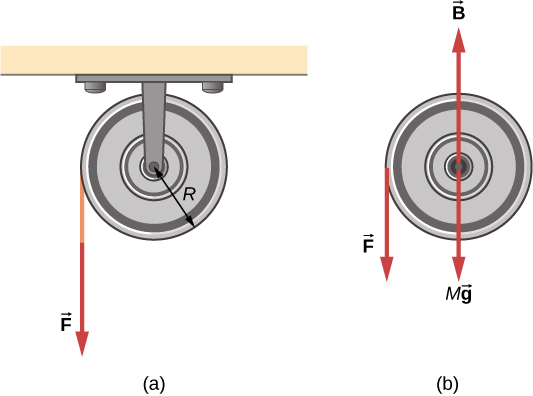Figure 10.40 (a) A string is wrapped around a pulley of radius R. (b) The free-body diagram.

#### Strategy

Looking at the free-body diagram, we see that neither $\overset{\to }{B}$, the force on the bearings of the pulley, nor$M\overset{\to }{g}$, the weight of the pulley, exerts a torque around the rotational axis, and therefore does no work on the pulley. As the pulley rotates through an angle $\theta ,$ $\overset{\to }{F}$ acts through a distance d such that $d=R\theta .$

#### Solution

Since the torque due to $\overset{\to }{F}$ has magnitude $\tau =RF$, we have

$W=\tau \theta =(FR)\theta =Fd.$

If the force on the string acts through a distance of 1.0 m, we have, from the work-energy theorem,

$\begin{array}{ccc}\hfill {W}_{AB}& =\hfill & {K}_{B}-{K}_{A}\hfill \\ \hfill Fd& =\hfill & \frac{1}{2}I{\omega }^{2}-0\hfill \\ \hfill (50.0\,\text{N})(1.0\,\text{m})& =\hfill & \frac{1}{2}(2.5\,×\,{10}^{-3}{\text{kg-m}}^{2}){\omega }^{2}.\hfill \end{array}$

Solving for $\omega$, we obtain

$\omega =200.0\,\text{rad}\text{/}\text{s}.$

### Power for Rotational Motion

Power always comes up in the discussion of applications in engineering and physics. Power for rotational motion is equally as important as power in linear motion and can be derived in a similar way as in linear motion when the force is a constant. The linear power when the force is a constant is $P=\overset{\to }{F}·\overset{\to }{v}$. If the net torque is constant over the angular displacement, (Figure) simplifies and the net torque can be taken out of the integral. In the following discussion, we assume the net torque is constant. We can apply the definition of power derived in Power to rotational motion. From Work and Kinetic Energy, the instantaneous power (or just power) is defined as the rate of doing work,

$P=\frac{dW}{dt}.$

If we have a constant net torque, (Figure) becomes $W=\tau \theta$ and the power is

$P=\frac{dW}{dt}=\frac{d}{dt}(\tau \theta )=\tau \frac{d\theta }{dt}$

or

$P=\tau \omega .$

### Example

#### Torque on a Boat Propeller

A boat engine operating at $9.0\,×\,{10}^{4}\,\text{W}$ is running at 300 rev/min. What is the torque on the propeller shaft?

#### Strategy

We are given the rotation rate in rev/min and the power consumption, so we can easily calculate the torque.

#### Solution

$300.0\,\text{rev/min}=31.4\,\text{rad/s;}$
$\tau =\frac{P}{\omega }=\frac{9.0\,×\,{10}^{4}\text{N}·\text{m}\text{/}\text{s}}{31.4\,\text{rad}\text{/}\text{s}}=2864.8\,\text{N}·\text{m}.$

#### Significance

It is important to note the radian is a dimensionless unit because its definition is the ratio of two lengths. It therefore does not appear in the solution.

A constant torque of $500\,\text{kN}·\text{m}$ is applied to a wind turbine to keep it rotating at 6 rad/s. What is the power required to keep the turbine rotating?

### Rotational and Translational Relationships Summarized

The rotational quantities and their linear analog are summarized in three tables. (Figure) summarizes the rotational variables for circular motion about a fixed axis with their linear analogs and the connecting equation, except for the centripetal acceleration, which stands by itself. (Figure) summarizes the rotational and translational kinematic equations. (Figure) summarizes the rotational dynamics equations with their linear analogs.

Rotational and Translational Variables: Summary
Rotational Translational Relationship
$\theta$ $x$ $\theta =\frac{s}{r}$
$\omega$ ${v}_{t}$ $\omega =\frac{{v}_{t}}{r}$
$\alpha$ ${a}_{\text{t}}$ $\alpha =\frac{{a}_{\text{t}}}{r}$
${a}_{\text{c}}$ ${a}_{\text{c}}=\frac{{v}_{\text{t}}^{2}}{r}$
Rotational and Translational Kinematic Equations: Summary
Rotational Translational
${\theta }_{\text{f}}={\theta }_{0}+\overset{–}{\omega }t$ $x={x}_{0}+\overset{–}{v}t$
${\omega }_{\text{f}}={\omega }_{0}+\alpha t$ ${v}_{\text{f}}={v}_{0}+at$
${\theta }_{\text{f}}={\theta }_{0}+{\omega }_{0}t+\frac{1}{2}\alpha {t}^{2}$ ${x}_{\text{f}}={x}_{0}+{v}_{0}t+\frac{1}{2}a{t}^{2}$
${\omega }_{\text{f}}^{2}={\omega }^{2}{}_{0}+2\alpha (\text{Δ}\theta )$ ${v}_{\text{f}}^{2}={v}^{2}{}_{0}+2a(\text{Δ}x)$
Rotational and Translational Equations: Dynamics
Rotational Translational
$I=\sum _{i}{m}_{i}{r}_{i}^{2}$ m
$K=\frac{1}{2}I{\omega }^{2}$ $K=\frac{1}{2}m{v}^{2}$
$\sum _{i}{\tau }_{i}=I\alpha$ $\sum _{i}{\overset{\to }{F}}_{i}=m\overset{\to }{a}$
${W}_{AB}=\underset{{\theta }_{A}}{\overset{{\theta }_{B}}{\int }}(\sum _{i}{\tau }_{i})d\theta$ $W=\int \overset{\to }{F}·d\overset{\to }{s}$
$P=\tau \omega$ $P=\overset{\to }{F}·\overset{\to }{v}$

### Summary

• The incremental work dW in rotating a rigid body about a fixed axis is the sum of the torques about the axis times the incremental angle $d\theta$.
• The total work done to rotate a rigid body through an angle $\theta$ about a fixed axis is the sum of the torques integrated over the angular displacement. If the torque is a constant as a function of $\theta$, then ${W}_{AB}=\tau ({\theta }_{B}-{\theta }_{A})$.
• The work-energy theorem relates the rotational work done to the change in rotational kinetic energy: ${W}_{AB}={K}_{B}-{K}_{A}$ where $K=\frac{1}{2}I{\omega }^{2}.$
• The power delivered to a system that is rotating about a fixed axis is the torque times the angular velocity, $P=\tau \omega$.

### Key Equations

 Angular position $\theta =\frac{s}{r}$ Angular velocity $\omega =\underset{\text{Δ}t\to 0}{\text{lim}}\frac{\text{Δ}\theta }{\text{Δ}t}=\frac{d\theta }{dt}$ Tangential speed ${v}_{\text{t}}=r\omega$ Angular acceleration $\alpha =\underset{\text{Δ}t\to 0}{\text{lim}}\frac{\text{Δ}\omega }{\text{Δ}t}=\frac{d\omega }{dt}=\frac{{d}^{2}\theta }{d{t}^{2}}$ Tangential acceleration ${a}_{\text{t}}=r\alpha$ Average angular velocity $\overset{–}{\omega }=\frac{{\omega }_{0}+{\omega }_{\text{f}}}{2}$ Angular displacement ${\theta }_{\text{f}}={\theta }_{0}+\overset{–}{\omega }t$ Angular velocity from constant angular acceleration ${\omega }_{\text{f}}={\omega }_{0}+\alpha t$ Angular velocity from displacement and constant angular acceleration ${\theta }_{\text{f}}={\theta }_{0}+{\omega }_{0}t+\frac{1}{2}\alpha {t}^{2}$ Change in angular velocity ${\omega }_{\text{f}}^{2}={\omega }_{0}^{2}+2\alpha (\text{Δ}\theta )$ Total acceleration $\overset{\to }{a}={\overset{\to }{a}}_{\text{c}}+{\overset{\to }{a}}_{\text{t}}$ Rotational kinetic energy $K=\frac{1}{2}(\sum _{j}{m}_{j}{r}_{j}^{2}){\omega }^{2}$ Moment of inertia $I=\sum _{j}{m}_{j}{r}_{j}^{2}$ Rotational kinetic energy in terms of the moment of inertia of a rigid body $K=\frac{1}{2}I{\omega }^{2}$ Moment of inertia of a continuous object $I=\int {r}^{2}dm$ Parallel-axis theorem ${I}_{\text{parallel-axis}}={I}_{\text{initial}}+m{d}^{2}$ Moment of inertia of a compound object ${I}_{\text{total}}=\sum _{i}{I}_{i}$ Torque vector $\overset{\to }{\tau }=\overset{\to }{r}\,×\,\overset{\to }{F}$ Magnitude of torque $|\overset{\to }{\tau }|={r}_{\perp }F$ Total torque ${\tau }_{\text{net}}=\sum _{i}|{\tau }_{i}|$ Newton’s second law for rotation $\sum _{i}{\tau }_{i}=I\alpha$ Incremental work done by a torque $dW=(\sum _{i}{\tau }_{i})d\theta$ Work-energy theorem ${W}_{AB}={K}_{B}-{K}_{A}$ Rotational work done by net force ${W}_{AB}=\underset{{\theta }_{A}}{\overset{{\theta }_{B}}{\int }}(\sum _{i}{\tau }_{i})d\theta$ Rotational power $P=\tau \omega$

### Problems

A wind turbine rotates at 20 rev/min. If its power output is 2.0 MW, what is the torque produced on the turbine from the wind?

A clay cylinder of radius 20 cm on a potter’s wheel spins at a constant rate of 10 rev/s. The potter applies a force of 10 N to the clay with his hands where the coefficient of friction is 0.1 between his hands and the clay. What is the power that the potter has to deliver to the wheel to keep it rotating at this constant rate?

A uniform cylindrical grindstone has a mass of 10 kg and a radius of 12 cm. (a) What is the rotational kinetic energy of the grindstone when it is rotating at $1.5\,×\,{10}^{3}\text{rev}\text{/}\text{min}?$ (b) After the grindstone’s motor is turned off, a knife blade is pressed against the outer edge of the grindstone with a perpendicular force of 5.0 N. The coefficient of kinetic friction between the grindstone and the blade is 0.80. Use the work energy theorem to determine how many turns the grindstone makes before it stops.

A uniform disk of mass 500 kg and radius 0.25 m is mounted on frictionless bearings so it can rotate freely around a vertical axis through its center (see the following figure). A cord is wrapped around the rim of the disk and pulled with a force of 10 N. (a) How much work has the force done at the instant the disk has completed three revolutions, starting from rest? (b) Determine the torque due to the force, then calculate the work done by this torque at the instant the disk has completed three revolutions? (c) What is the angular velocity at that instant? (d) What is the power output of the force at that instant?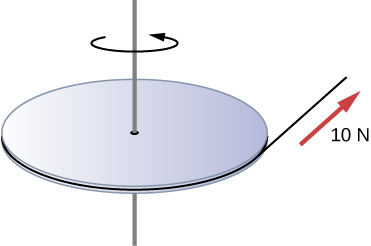A propeller is accelerated from rest to an angular velocity of 1000 rev/min over a period of 6.0 seconds by a constant torque of $2.0\,×\,{10}^{3}\text{N}·\text{m}$. (a) What is the moment of inertia of the propeller? (b) What power is being provided to the propeller 3.0 s after it starts rotating?

A sphere of mass 1.0 kg and radius 0.5 m is attached to the end of a massless rod of length 3.0 m. The rod rotates about an axis that is at the opposite end of the sphere (see below). The system rotates horizontally about the axis at a constant 400 rev/min. After rotating at this angular speed in a vacuum, air resistance is introduced and provides a force $0.15\,\text{N}$ on the sphere opposite to the direction of motion. What is the power provided by air resistance to the system 100.0 s after air resistance is introduced?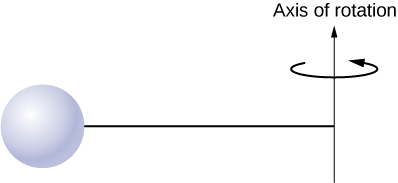A uniform rod of length L and mass M is held vertically with one end resting on the floor as shown below. When the rod is released, it rotates around its lower end until it hits the floor. Assuming the lower end of the rod does not slip, what is the linear velocity of the upper end when it hits the floor?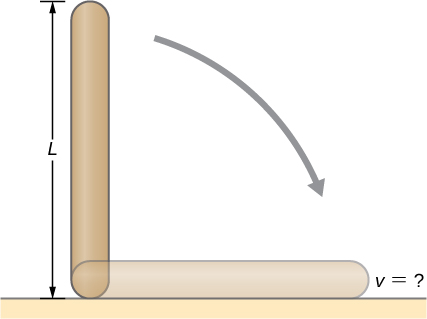An athlete in a gym applies a constant force of 50 N to the pedals of a bicycle to keep the rotation rate of the wheel at 10 rev/s. The length of the pedal arms is 30 cm. What is the power delivered to the bicycle by the athlete?

A 2-kg block on a frictionless inclined plane at $40\text{°}$ has a cord attached to a pulley of mass 1 kg and radius 20 cm (see the following figure). (a) What is the acceleration of the block down the plane? (b) What is the work done by the gravitational force to move the block 50 cm?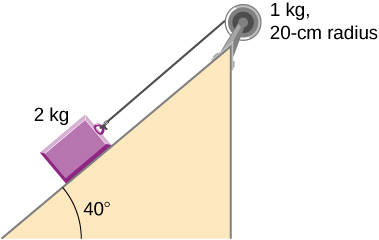Small bodies of mass ${m}_{1}\,\text{and}\,{m}_{2}$ are attached to opposite ends of a thin rigid rod of length L and mass M. The rod is mounted so that it is free to rotate in a horizontal plane around a vertical axis (see below). What distance d from ${m}_{1}$ should the rotational axis be so that a minimum amount of work is required to set the rod rotating at an angular velocity $\omega ?$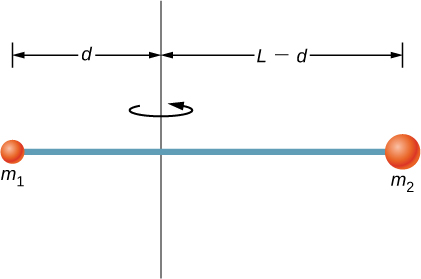A cyclist is riding such that the wheels of the bicycle have a rotation rate of 3.0 rev/s. If the cyclist brakes such that the rotation rate of the wheels decrease at a rate of $0.3\,\text{rev}\text{/}{\text{s}}^{2}$, how long does it take for the cyclist to come to a complete stop?

Calculate the angular velocity of the orbital motion of Earth around the Sun.

A phonograph turntable rotating at 33 1/3 rev/min slows down and stops in 1.0 min. (a) What is the turntable’s angular acceleration assuming it is constant? (b) How many revolutions does the turntable make while stopping?

With the aid of a string, a gyroscope is accelerated from rest to 32 rad/s in 0.40 s under a constant angular acceleration. (a) What is its angular acceleration in ${\text{rad/s}}^{2}$? (b) How many revolutions does it go through in the process?

Suppose a piece of dust has fallen on a CD. If the spin rate of the CD is 500 rpm, and the piece of dust is 4.3 cm from the center, what is the total distance traveled by the dust in 3 minutes? (Ignore accelerations due to getting the CD rotating.)

A system of point particles is rotating about a fixed axis at 4 rev/s. The particles are fixed with respect to each other. The masses and distances to the axis of the point particles are ${m}_{1}=0.1\,\text{kg},{r}_{1}=0.2\,\text{m}$, ${m}_{2}=0.05\,\text{kg},{r}_{2}=0.4\,\text{m}$, ${m}_{3}=0.5\,\text{kg},{r}_{3}=0.01\,\text{m}$. (a) What is the moment of inertia of the system? (b) What is the rotational kinetic energy of the system?

Calculate the moment of inertia of a skater given the following information. (a) The 60.0-kg skater is approximated as a cylinder that has a 0.110-m radius. (b) The skater with arms extended is approximated by a cylinder that is 52.5 kg, has a 0.110-m radius, and has two 0.900-m-long arms which are 3.75 kg each and extend straight out from the cylinder like rods rotated about their ends.

A stick of length 1.0 m and mass 6.0 kg is free to rotate about a horizontal axis through the center. Small bodies of masses 4.0 and 2.0 kg are attached to its two ends (see the following figure). The stick is released from the horizontal position. What is the angular velocity of the stick when it swings through the vertical?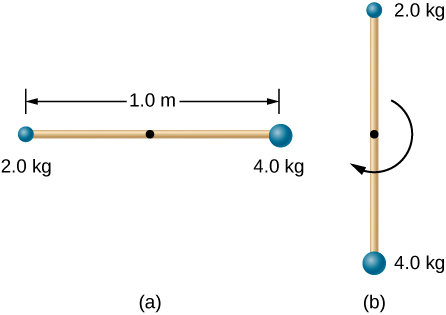A pendulum consists of a rod of length 2 m and mass 3 kg with a solid sphere of mass 1 kg and radius 0.3 m attached at one end. The axis of rotation is as shown below. What is the angular velocity of the pendulum at its lowest point if it is released from rest at an angle of $30\text{°}?$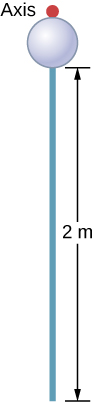Calculate the torque of the 40-N force around the axis through O and perpendicular to the plane of the page as shown below.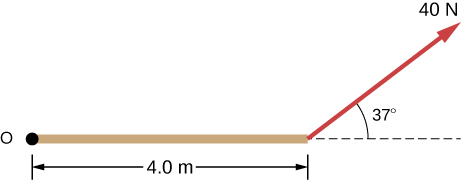Two children push on opposite sides of a door during play. Both push horizontally and perpendicular to the door. One child pushes with a force of 17.5 N at a distance of 0.600 m from the hinges, and the second child pushes at a distance of 0.450 m. What force must the second child exert to keep the door from moving? Assume friction is negligible.

The force of $20\hat{j}\text{N}$ is applied at $\overset{\to }{r}=(4.0\hat{i}-2.0\hat{j})\,\text{m}$. What is the torque of this force about the origin?

An automobile engine can produce 200 N$·$ m of torque. Calculate the angular acceleration produced if 95.0% of this torque is applied to the drive shaft, axle, and rear wheels of a car, given the following information. The car is suspended so that the wheels can turn freely. Each wheel acts like a 15.0-kg disk that has a 0.180-m radius. The walls of each tire act like a 2.00-kg annular ring that has inside radius of 0.180 m and outside radius of 0.320 m. The tread of each tire acts like a 10.0-kg hoop of radius 0.330 m. The 14.0-kg axle acts like a rod that has a 2.00-cm radius. The 30.0-kg drive shaft acts like a rod that has a 3.20-cm radius.

A grindstone with a mass of 50 kg and radius 0.8 m maintains a constant rotation rate of 4.0 rev/s by a motor while a knife is pressed against the edge with a force of 5.0 N. The coefficient of kinetic friction between the grindstone and the blade is 0.8. What is the power provided by the motor to keep the grindstone at the constant rotation rate?

### Challenge Problems

The angular acceleration of a rotating rigid body is given by $\alpha =(2.0-3.0t)\,\text{rad}\text{/}{\text{s}}^{2}$. If the body starts rotating from rest at $t=0$, (a) what is the angular velocity? (b) Angular position? (c) What angle does it rotate through in 10 s? (d) Where does the vector perpendicular to the axis of rotation indicating $0\text{°}$ at $t=0$ lie at $t=10\,\text{s}$?

Earth’s day has increased by 0.002 s in the last century. If this increase in Earth’s period is constant, how long will it take for Earth to come to rest?

A disk of mass m, radius R, and area A has a surface mass density $\sigma =\frac{mr}{AR}$ (see the following figure). What is the moment of inertia of the disk about an axis through the center?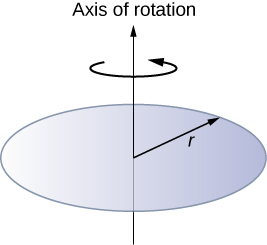Zorch, an archenemy of Rotation Man, decides to slow Earth’s rotation to once per 28.0 h by exerting an opposing force at and parallel to the equator. Rotation Man is not immediately concerned, because he knows Zorch can only exert a force of $4.00\,×\,1{0}^{7}\text{N}$ (a little greater than a Saturn V rocket’s thrust). How long must Zorch push with this force to accomplish his goal? (This period gives Rotation Man time to devote to other villains.)

A cord is wrapped around the rim of a solid cylinder of radius 0.25 m, and a constant force of 40 N is exerted on the cord shown, as shown in the following figure. The cylinder is mounted on frictionless bearings, and its moment of inertia is $6.0\,\text{kg}·{\text{m}}^{2}$. (a) Use the work energy theorem to calculate the angular velocity of the cylinder after 5.0 m of cord have been removed. (b) If the 40-N force is replaced by a 40-N weight, what is the angular velocity of the cylinder after 5.0 m of cord have unwound?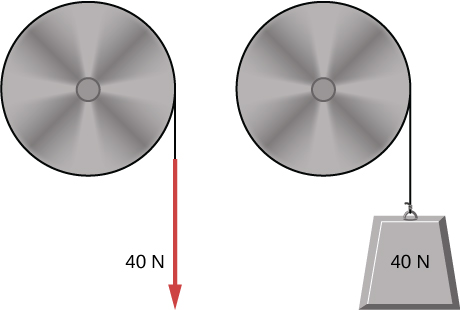### Glossary

rotational work
work done on a rigid body due to the sum of the torques integrated over the angle through with the body rotates
work-energy theorem for rotation
the total rotational work done on a rigid body is equal to the change in rotational kinetic energy of the body